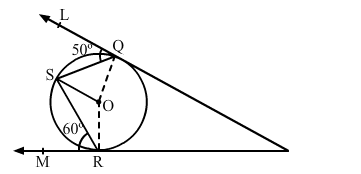# In the given figure, O is the centre of a circle; PQL and PRM are the tangents at the points Q and R respectively,`
Question:

In the given figure, O is the centre of a circle; PQL and PRM are the tangents at the points Q and R respectively, and is a point on the circle, such that ∠SQL = 50° and ∠SRM = 60°. Find ∠QSR.(a) 40°
(b) 50°
(c) 60°
(d) 70°

Solution:

(d) $70^{\circ}$

$P Q L$ is a tangent $O Q$ is the radius; so, $\angle O Q L=90^{\circ}$.

$\therefore \angle \mathrm{OQS}=\left(90^{0}-50^{0}\right)=40^{\circ}$

Now, OQ $=$ OS (Ra dius of the same circle)

$\Rightarrow \angle \mathrm{OSQ}=\angle \mathrm{OQS}=40^{\circ}$

Similarly, $\angle \mathrm{ORS}=\left(90^{0}-60^{\circ}\right)=30^{0}$

And , OR $=$ OS (Radius of the same circle)

$\Rightarrow \angle O S R=\angle O R S=30^{\circ}$

$\therefore \angle \mathrm{QSR}=\angle \mathrm{OSQ}+\angle \mathrm{OSR}$

$\Rightarrow \angle \mathrm{QSR}=\left(40^{0}+30^{0}\right)$

$\Rightarrow \angle \mathrm{QSR}=70^{\circ}$